# Introduction

The RCM package combines unconstrained and constrained ordination of microbiome read count data into a single package. The package functions allow fitting of the ordination model, plotting it and performing diagnostic checks.

# Installation

The package can be installed and loaded using the following commands:

# library(BiocManager)
# BiocManager::install("RCM")
library(RCM)
## Registered S3 methods overwritten by 'ggplot2':
##   method         from
##   [.quosures     rlang
##   c.quosures     rlang
##   print.quosures rlang
## Registered S3 method overwritten by 'xts':
##   method     from
##   as.zoo.xts zoo
## Registered S3 method overwritten by 'quantmod':
##   method            from
##   as.zoo.data.frame zoo
cat("RCM package version", as.character(packageVersion("RCM")), "\n")
## RCM package version 1.0.0

## Dataset

As example data we use a study on the microbiome of colorectal cancer patients “Potential of fecal microbiota for early-stage detection of colorectal cancer” (2014) by Zeller et al..

data(Zeller)
library(phyloseq)

## Unconstrained RCM

### Fitting the unconstrained RCM

The unconstrained RC(M) method represents all variability present in the data, regardless of covariate information. It should be used as a first step in an exploratory analysis. The RCM function is the front end function for this purpose, behind it is the RCM_NB function that does the hard work but requires numeric matrix imputs. We first fit a model with two dimensions,

ZellerRCM2 = RCM(Zeller, k = 2, round = TRUE)

which took 0.7 minutes. Here we supplied a phyloseq object to the RCM function which is preferable. Alternatively one can also provide a count matrix with samples in the rows and taxa in the columns, but then all plotting variables should be supplied manually as vectors.

By default, only 2 dimensions are fitted, but more can be requested throught the k argument.

ZellerRCM3 = RCM(Zeller, k = 3, round = TRUE)

The total runtime for all dimensions combined was 1.8 minutes.

#### Conditioning

In order to condition on certain variables, supply their names to the confounders argument. Here we condition on the country variable

ZellerRCM2cond = RCM(Zeller, k = 2, round = TRUE, confounders = c("Country"))

Conditioning can be applied for unconstrained as well as constrained RCM, the ensuing plots have exactly the same interpretation as before, only now the variability due to the confounding variable has been filtered out.

### Plotting the uconstrained RCM

#### Monoplots

To plot only samples or taxa, specify the plotType argument in the generic plot function.

plot(ZellerRCM2, plotType = "samples")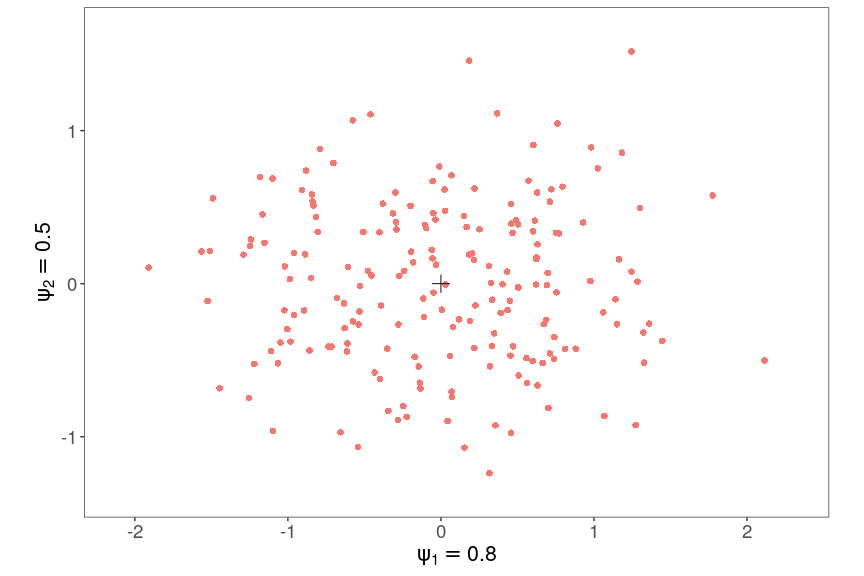No clear signal is present at first sight. Note also that the plot is rectangular according to the values of the importance parameters $$\psi$$. In order to truthfully represent the distances between samples all axis must be on the same scale. We can add a colour code for the cancer diagnosis contained in the phyloseq object.

plot(ZellerRCM2, plotType = "samples", samColour = "Diagnosis")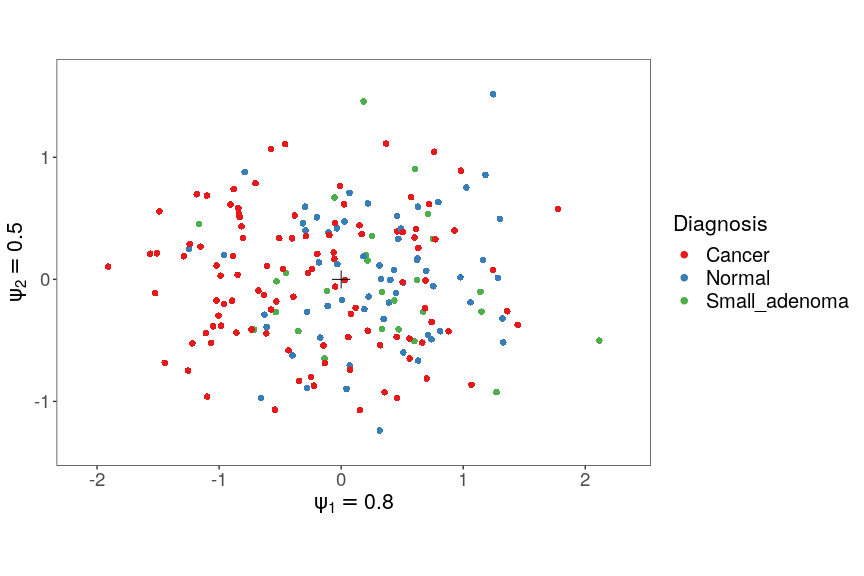It is clear that some of the variability of the samples is explained by the cancer status of the patients.

We can also add a richness measure as a colur, see ?phyloseq::estimate_richness for a list of available richness measures. Here we plot the Shannon diversity

plot(ZellerRCM2, plotType = "samples", samColour = "Shannon")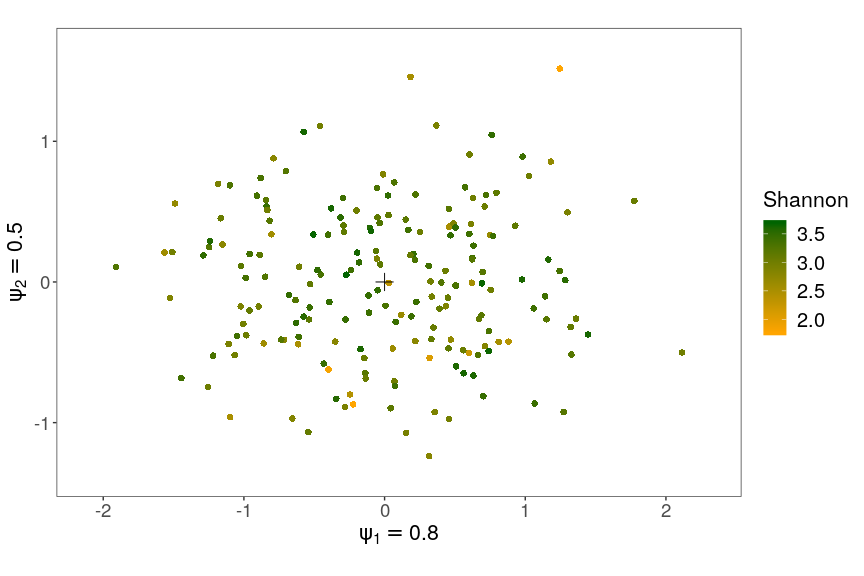In order to plot only the species, modify the plotType argument.

plot(ZellerRCM2, plotType = "species")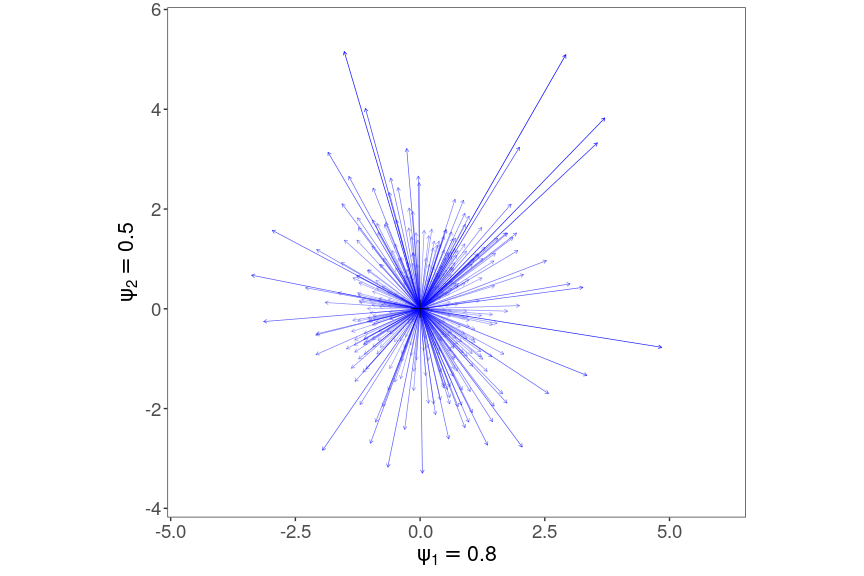The researchers found that species from the Fusobacteria genus are associated with cancer. We can plot only these species using a regular expression.

plot(ZellerRCM2, plotType = "species", taxRegExp = "Fusobacter", taxLabels = TRUE)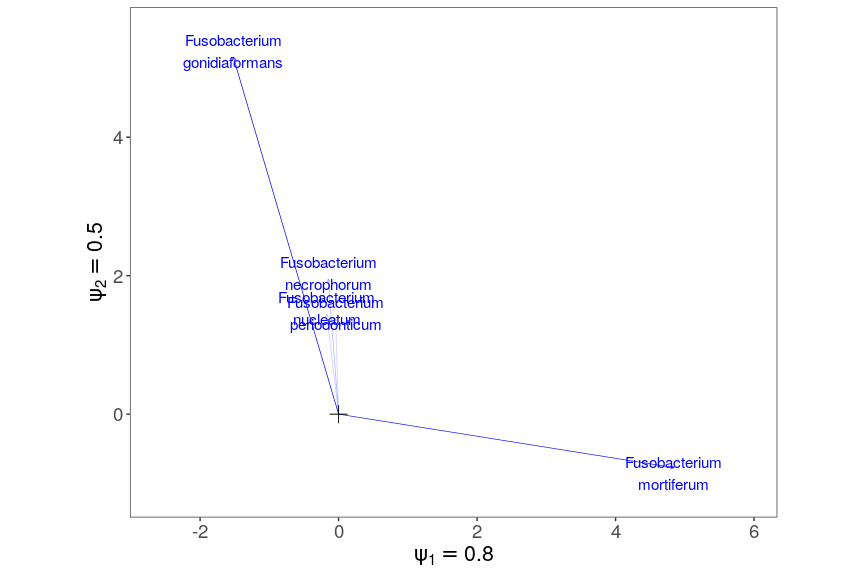It is clear that these Fusobacterium species behave very differently between the species. We can also colour the species plots by phylogenetic level, e.g. order level, if available in the dataset.

plot(ZellerRCM2, plotType = "species", taxLabels = TRUE, taxCol = "Order")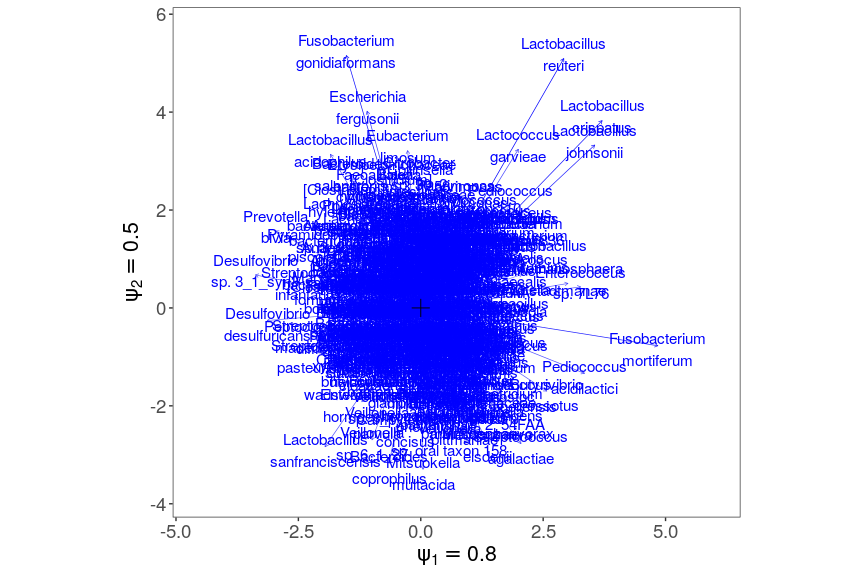#### Biplots

Finally we can combine both plots into an interpretable biplot, which is the default for unconstrained RC(M). To avoid overplotting we only show the taxa with the 10 most important departures from independence.

plot(ZellerRCM2, taxNum = 10, samColour = "Diagnosis")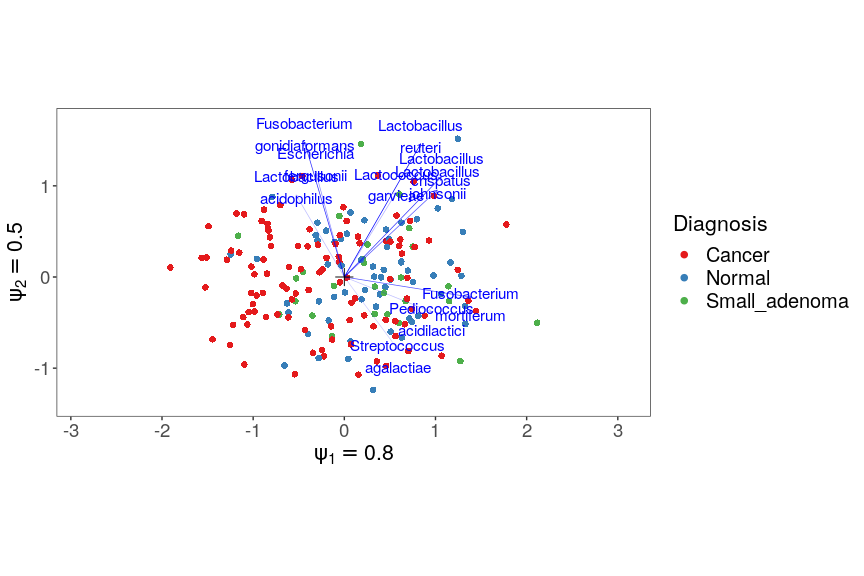Samples are represented by dots, taxa by arrows. Both represent vectors with the origin as starting point.

Valid interpretatations are the following:

• Samples (endpoints of sample vectors, the red dots) close together depart from independence in a similar way
• The orthogonal projection of the taxon arrows on the sample arrows are proportional to the departure from independence of that taxon in that sample on the log scale, in the first two dimensions. For example Fusobacterium mortiferum is more abundant than average in samples on the left side of the plot, and more abundant in samples on the right side.
• The importance parameters $$\psi$$ shown for every axis reflect the relative importance of the dimensions

Distances between endpoints of taxon vectors are meaningless.

We can also graphically highlight the departure from independence for a particular taxon and sample using the addOrthProjection function:

tmpPlot = plot(ZellerRCM2, taxNum = 10, samColour = "Diagnosis", returnCoords = TRUE)
addOrthProjection(tmpPlot, species = "Alloprevotella tannerae", sample = c(-1.2,
1.5))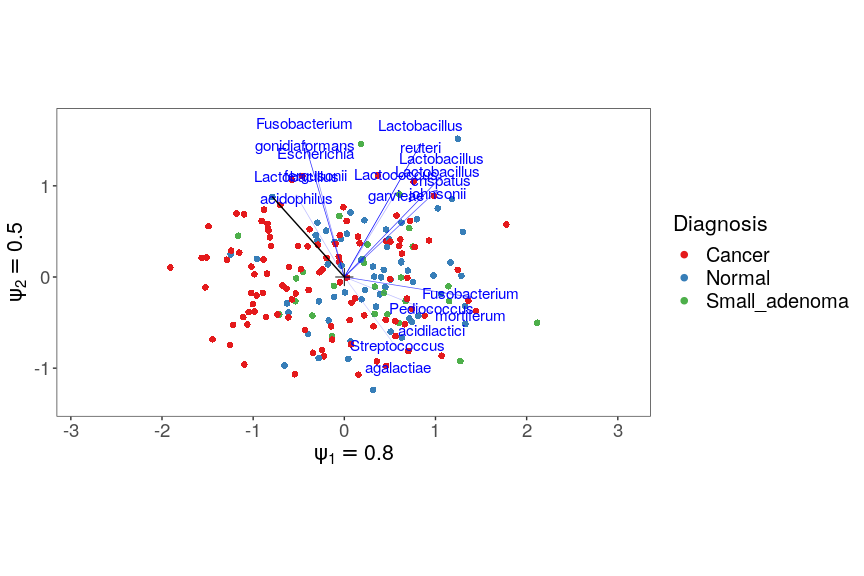The projection of the species vector is graphically shown here, the orange bar representing the extent of the departure from independence. Note that we providid the exact taxon name, and approximate sample coordinates visually derived from the graph, but any combination of both is possible.

We can then plot any combination of two dimensions we want, e.g. the first and the third.

plot(ZellerRCM3, Dim = c(1, 3), samColour = "Diagnosis", taxNum = 6)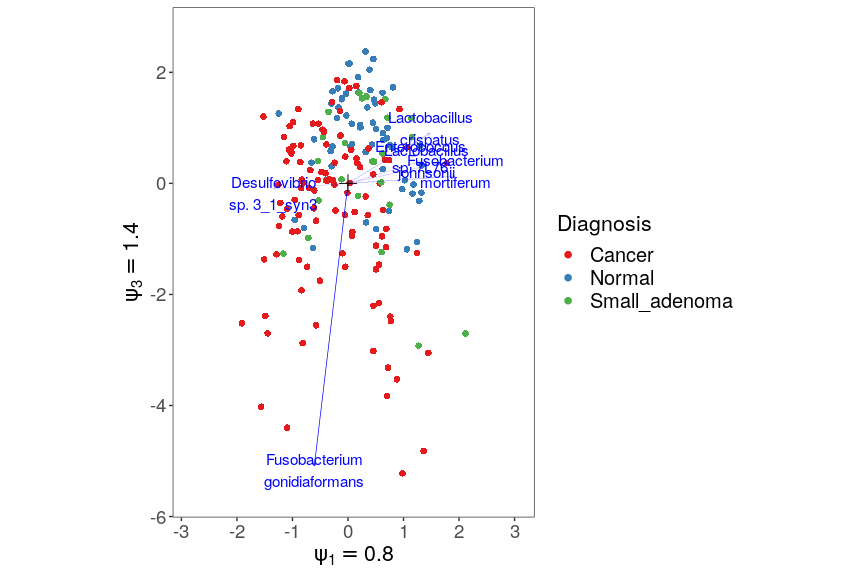The third dimension also correlates with a separation of cancer patients vs. healthy and small adenoma patients.

### Assessing the goodness of fit

Some taxa (or samples) may not follow a negative binomial distribution, or their departures from independence may not be appropriately represented in low dimensions. We visualize these taxa and samples through their deviances, which are the squared sums of their deviance residuals. This allows us to graphically identify taxa and samples that are poorly represented in the current ordination.

The deviances per sample can be plotted by just supplying the argument “Deviance” to samColour

plot(ZellerRCM2, plotType = "samples", samColour = "Deviance", samSize = 2.5)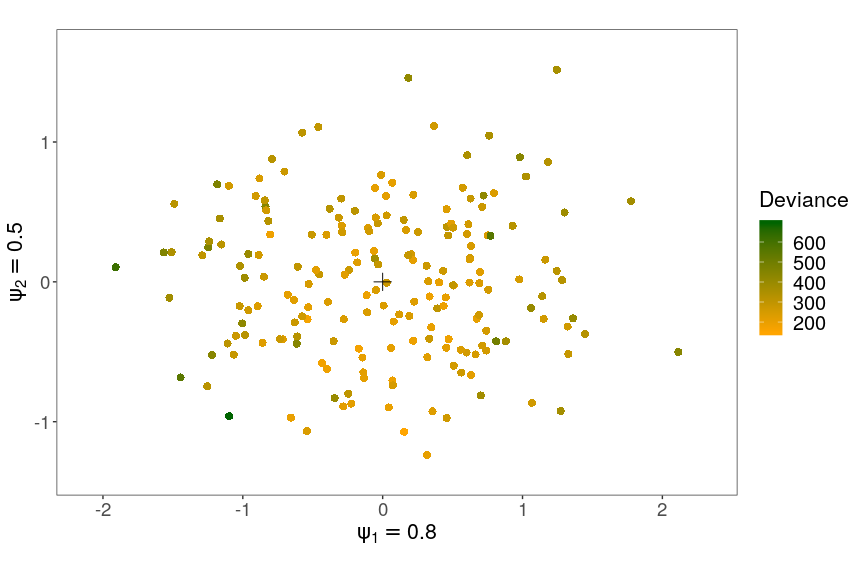Samples with the largest scores exhibit the poorest fit. This may indicate that samples with strong departures from independence acquire large scores, but still are not well represented in lower dimensions. Especially the one bottom right may be a problematic case.

The same principle can be applied to the taxa

plot(ZellerRCM3, plotType = "species", taxCol = "Deviance", samSize = 2.5, Dim = c(1,
2), arrowSize = 0.5)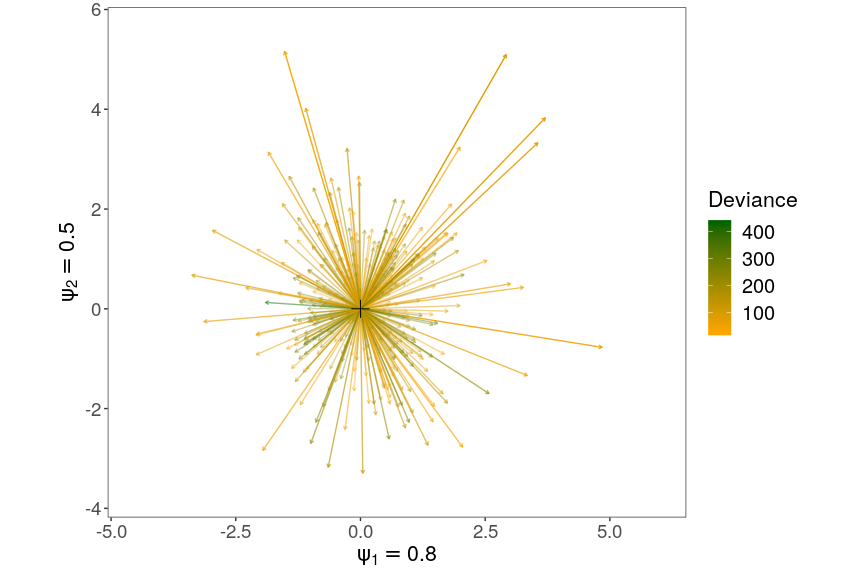For the taxa it appears to be the taxa with smaller scores are the more poorly fitted ones. Note that since the count table is not square, we cannot compare sample and taxon deviances. They have not been calculated based on the same number of taxa. Also, one cannot do chi-squared tests based on the deviances since this is not a classical regression model, but an overparametrized one.

## Constrained RCM

In this second step we look for the variability in the dataset explained by linear combinations of covariates that maximally separate the niches of the species. This should be done in a second step, and preferably only with variables that are believed to have an impact on the species’ abundances. Here we used the variables age, gender, BMI, country and diagnosis in the gradient. In this analysis all covariates values of a sample are projected onto a single scalar, the environmental score of this sample. The projection vector is called the environmental gradient, the magnitude of its components reveals the importance of each variable. The taxon-wise response functions then describe how the logged mean abundance depends on the environmental score.

### Fitting the constrained RCM model

In order to request a constrained RCM fit it suffises to supply the names of the constraining variables to the covariates argument. The shape of the response function can be either “linear”, “quadratic” or “nonparametric” and must be provided to the responseFun argument. Here we illustrate the use of linear and nonparametric response functions. Linear response functions may be too simplistic, they have the advantage of being easy to interpret (and plot). Non-parametric ones (based on splines) are more flexible but are harder to plot.

# Linear
ZellerRCM2constr = RCM(Zeller, k = 2, round = TRUE, covariates = c("Age", "Gender",
"BMI", "Country", "Diagnosis"), responseFun = "linear")
# Nonparametric
ZellerRCM2constrNonParam = RCM(Zeller, round = TRUE, k = 2, covariates = c("Age",
"Gender", "BMI", "Country", "Diagnosis"), responseFun = "nonparametric")

### Plotting the constrained RCM model

#### Monoplots

As before we can make monoplots of only samples or taxa. Starting with the samples

plot(ZellerRCM2constr, plotType = c("samples"))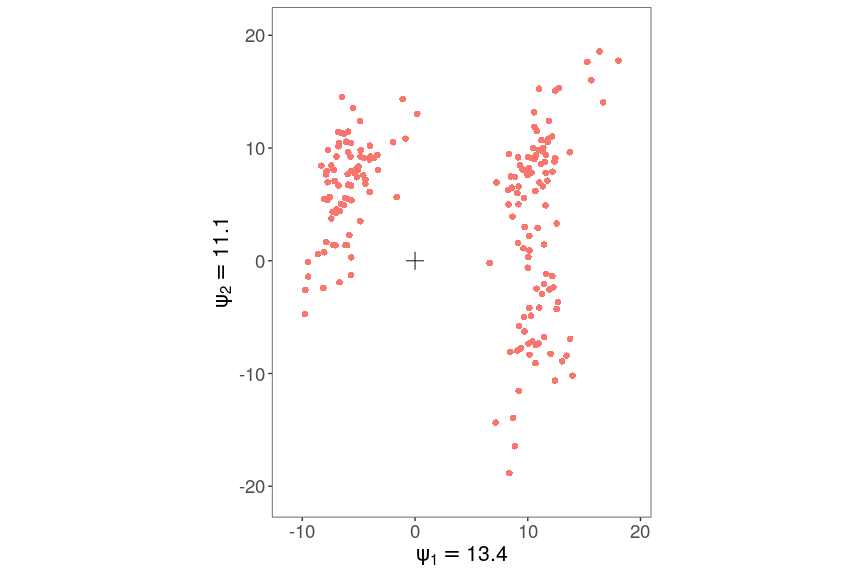we clearly see three groups of samples appearing.

plot(ZellerRCM2constr, plotType = c("samples"), samColour = "Diagnosis", samShape = "Country")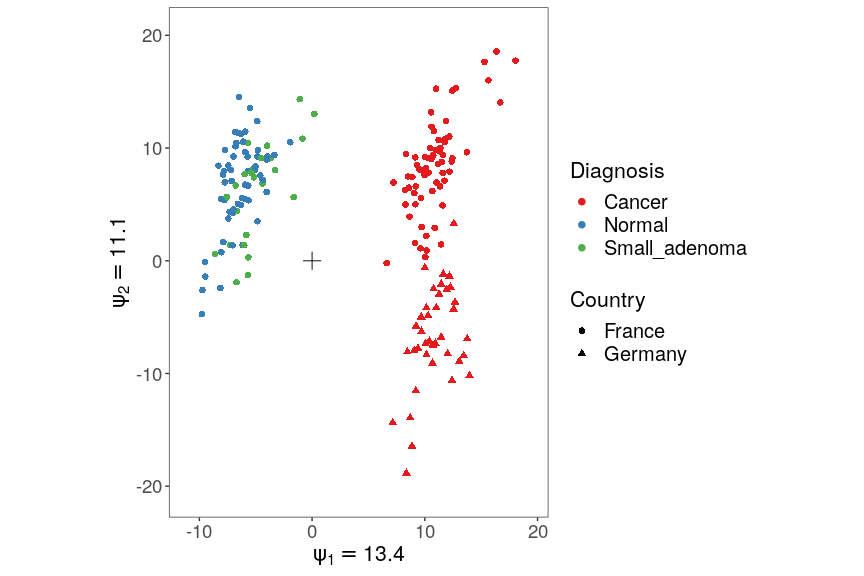One group are the healthy patients, the other two are cancer patients. The cancer patients are separated by country. Note that from Germany there are only cancer patients in this dataset. In case of non-parametric response functions, the samples mononoplot is not interpretable.

Unique to the constrained ordinations are monoplots of the variables. Do note however, that the axes have not been scaled, and trends on the x-and y-axis should be interpreted independently.

plot(ZellerRCM2constr, plotType = "variables")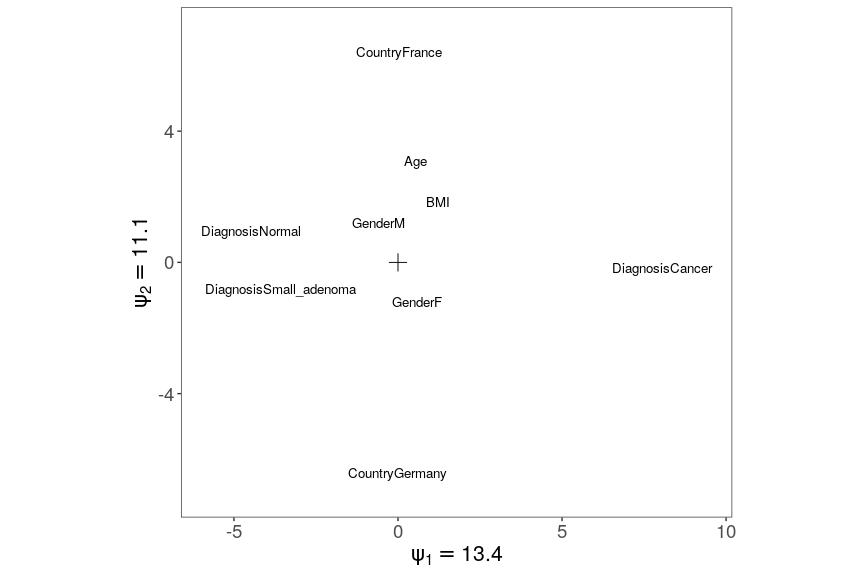Variables far away from the origin have a strong role in shaping the environmental gradient.

plot(ZellerRCM2constrNonParam, plotType = "variables")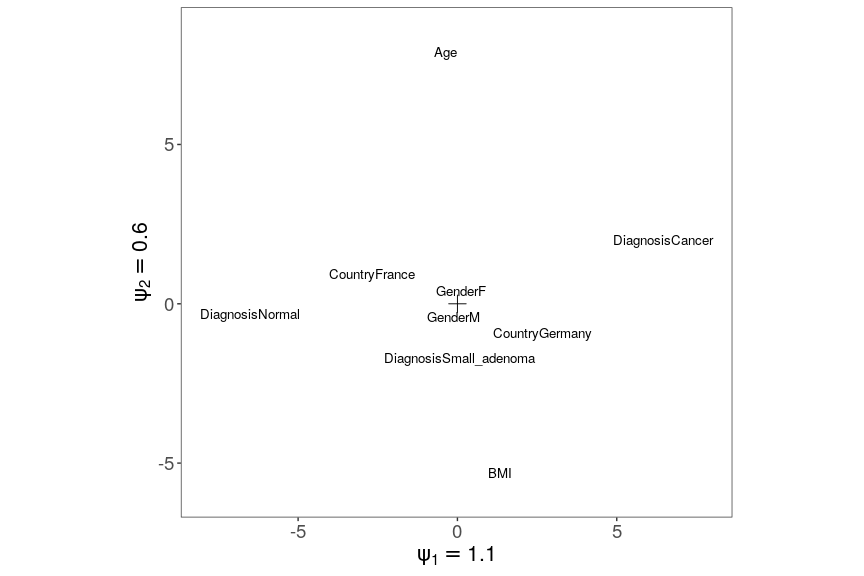The envrionmental gradients are quite different from the case with the linear response functions. Especially age is an important driver of the environmental gradient here. Still the results for cancer diagnosis, country and gender are similar to before.

#### Biplots

In the constrained case two different biplots are meaningful: sample-taxon biplots and variable-taxon biplots.

##### Sample-taxon biplot
plot(ZellerRCM2constr, plotType = c("species", "samples"))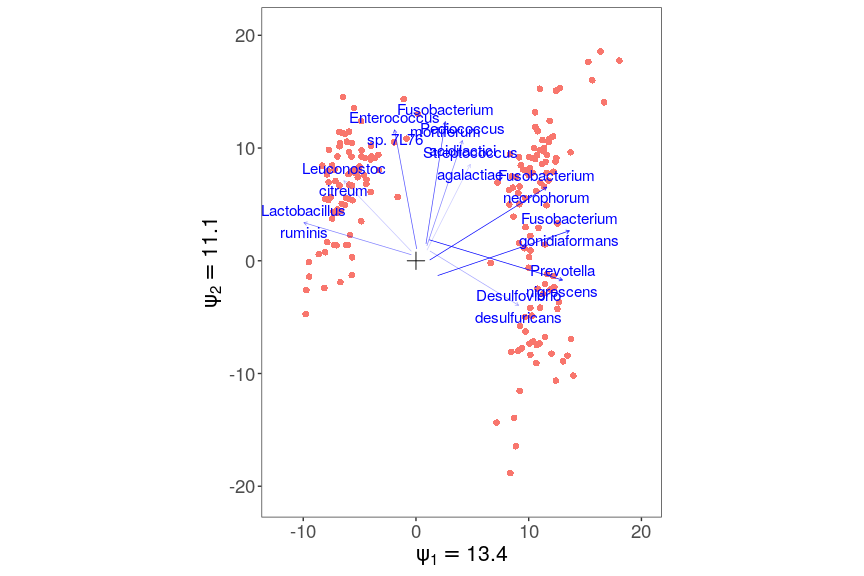The interpretation is similar as before: the orthogonal projection of a taxon’s arrow on a sample represents the departure from independence for that taxon in that sample, explained by environmental variables. New is also that the taxa arrows do not start from the origin, but all have their own starting point. This starting point represents the environmental scores for which there is no departure from independence. The direction of the arrow then represents in which direction of the environmental gradient its expected abundance increases. Again we can show this projection visually:

tmpPlot2 = plot(ZellerRCM2constr, plotType = c("species", "samples"), returnCoords = TRUE)
addOrthProjection(tmpPlot2, species = "Pseudomonas fluorescens", sample = c(-12,
7))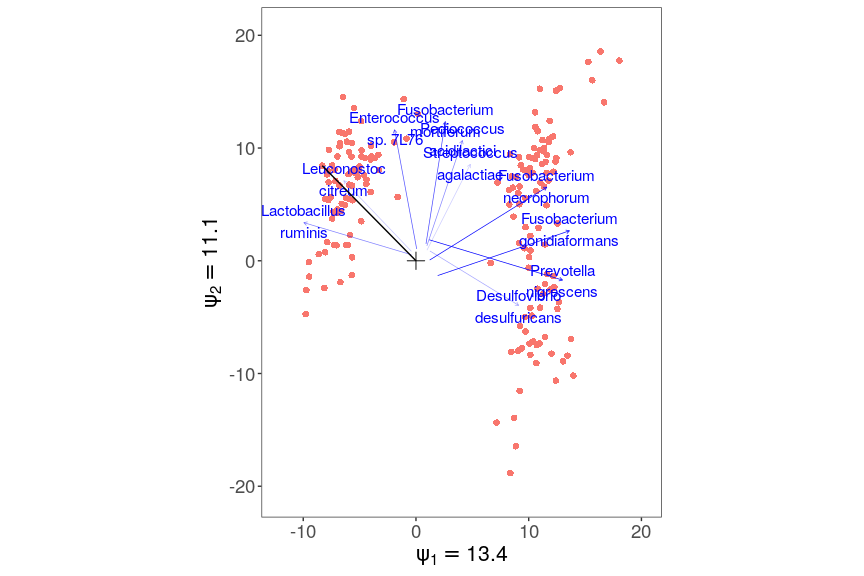Note that the projection bar does not start from the origin in this case either.

##### Variable-taxon biplot
plot(ZellerRCM2constr, plotType = c("species", "variables"))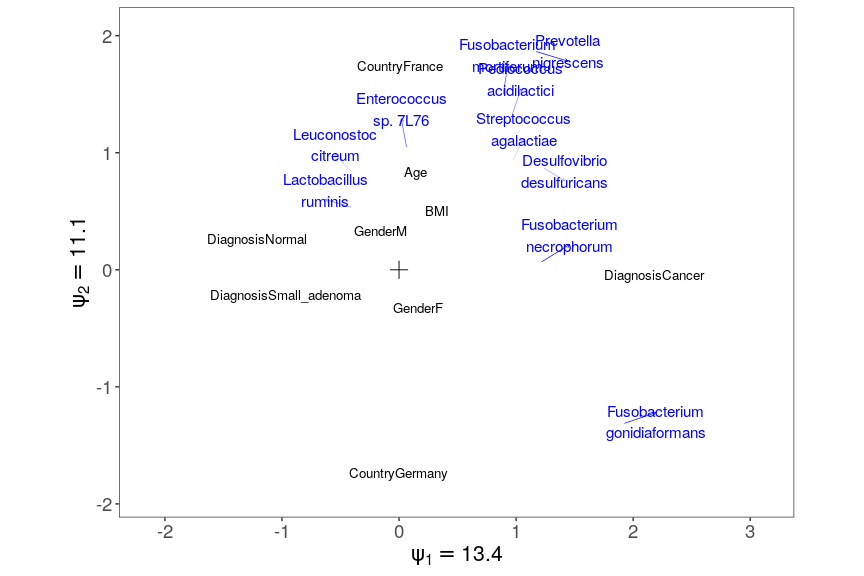The projection of species arrows on environmental variables (starting from the origin) represents the sensitivity of this taxon to changes in this variables. Note that the fact that the arrows of BMI and gender are of similar length indicates that one standard deviation in BMI has a similar effect to gender.

Also this interpretation we can show visually on the graph

tmpPlot3 = plot(ZellerRCM2constr, plotType = c("species", "variables"), returnCoords = TRUE)
addOrthProjection(tmpPlot3, species = "Pseudomonas fluorescens", variable = "DiagnosisSmall_adenoma")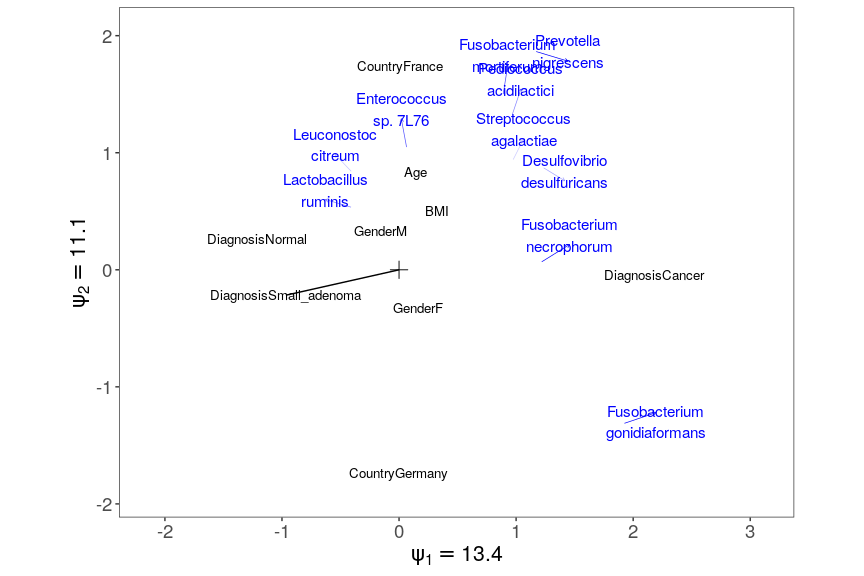We observe that country and diagnosis are the main drivers of the environmental gradient. We also see that the healthy status has a very similar to the small adenoma status, but that they are very different from cancer patients.

#### Triplot

Triplots combine all the three components in a single plot. This is the default behaviour of the plot function.

plot(ZellerRCM2constr)